# Heart of AlgebraPage 4

#### WATCH ALL SLIDES

Describing relationships shown graphically

Summarizing qualitative and quantitative data

Slide 22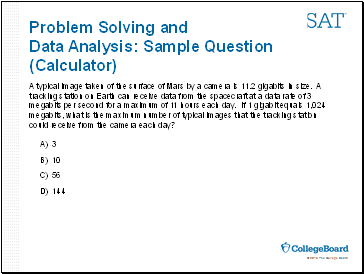Problem Solving and Data Analysis: Sample Question (Calculator)

A typical image taken of the surface of Mars by a camera is 11.2 gigabits in size. A tracking station on Earth can receive data from the spacecraft at a data rate of 3 megabits per second for a maximum of 11 hours each day. If 1 gigabit equals 1,024 megabits, what is the maximum number of typical images that the tracking station could receive from the camera each day?

A) 3

B) 10

C) 56

D) 144

Slide 23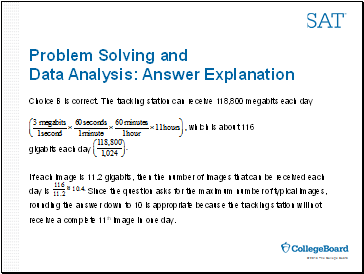Problem Solving and Data Analysis: Answer Explanation

Choice B is correct. The tracking station can receive 118,800 megabits each day

gigabits each day

If each image is 11.2 gigabits, then the number of images that can be received each day is Since the question asks for the maximum number of typical images, rounding the answer down to 10 is appropriate because the tracking station will not receive a complete 11th image in one day.

Slide 24## Connecting the Math Test with Classroom Instruction

Slide 25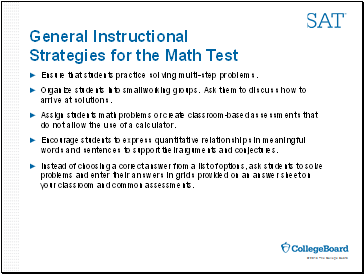General Instructional Strategies for the Math Test

Ensure that students practice solving multi-step problems.

Organize students into small working groups. Ask them to discuss how to arrive at solutions.

Assign students math problems or create classroom-based assessments that do not allow the use of a calculator.

Encourage students to express quantitative relationships in meaningful words and sentences to support their arguments and conjectures.

Slide 26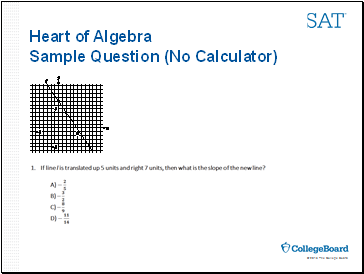Heart of Algebra Sample Question (No Calculator)

Slide 27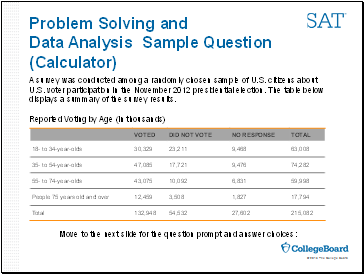Problem Solving and Data Analysis Sample Question (Calculator)

A survey was conducted among a randomly chosen sample of U.S. citizens about U.S. voter participation in the November 2012 presidential election. The table below displays a summary of the survey results.

Reported Voting by Age (in thousands)

Move to the next slide for the question prompt and answer choices:

Slide 28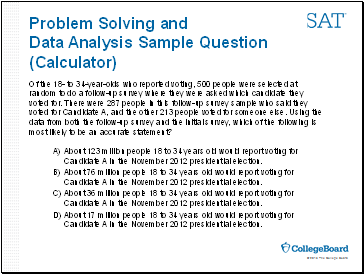Problem Solving and Data Analysis Sample Question (Calculator)

Go to page:
1  2  3  4  5  6  7